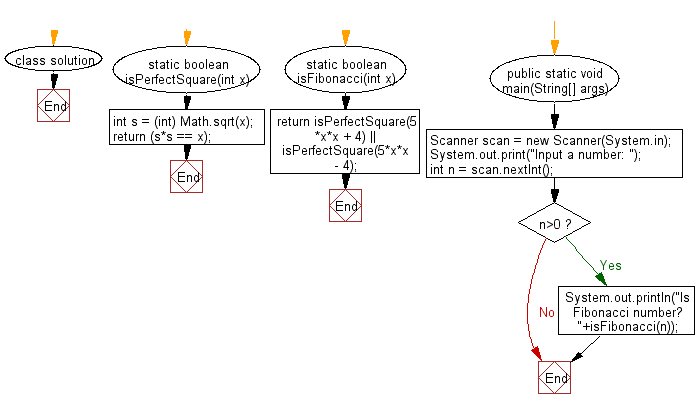﻿ Java Math Exercises: Check if a given number is Fibonacci number or not - w3resource# Java Math Exercises: Check if a given number is Fibonacci number or not

## Java Math Exercises: Exercise-27 with Solution

Write a Java program to check if a given number is Fibonacci number or not.

Sample Solution:

Java Code:

``````import java.util.*;

class solution {
static boolean isPerfectSquare(int x)
{
int s = (int) Math.sqrt(x);
return (s*s == x);
}

static boolean isFibonacci(int x)
{
return isPerfectSquare(5*x*x + 4) ||
isPerfectSquare(5*x*x - 4);
}

public static void main(String[] args)
{
Scanner scan = new Scanner(System.in);
System.out.print("Input a number: ");
int n = scan.nextInt();
if (n>0)
{
System.out.println("Is Fibonacci number? "+isFibonacci(n));
}
}
}
``````

Sample Output:

```Input a number:  55
Is Fibonacci number? true
```

Flowchart:Java Code Editor:

What is the difficulty level of this exercise?

﻿

## Java: Tips of the Day

Parsing dates:

```import java.io.*;
import java.util.*;
import java.text.*;

String s = "2001/09/23 14:39";

SimpleDateFormat formatter = new SimpleDateFormat ("yyyy/MM/dd H:mm");
Date d = formatter.parse(s, new ParsePosition(0));
```